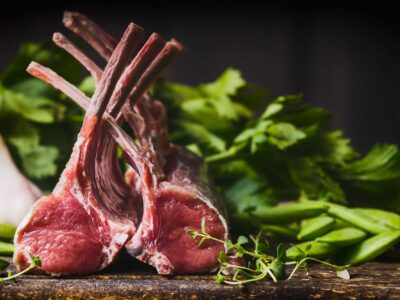Lamb Shoulder Chops Lamb\$12 jQuery(function(\$){ var price = '12'; currency = '\$'; /* \$('[name=quantity]').change(function(){ if (!(this.value < 1)) { var product_total = parseFloat(price * this.value); \$(this).closest('.woocom-list-content').find('.product_total_price .price .woocommerce-Price-amount').html(currency + product_total.toFixed(2)); } }); jQuery( '.variations_form' ).each( function() { jQuery(this).on( 'found_variation', function( event, variation ) { var price = variation.display_price; var quantity = \$(this).find('[name=quantity]').val(); if (!(quantity < 1)) { console.log(price); var product_total = parseFloat(price * quantity); \$(this).closest('.woocom-list-content').find('.product_total_price .price .woocommerce-Price-amount').html(currency + product_total.toFixed(2)); } }); });*/ }); 500g1 KG Lamb Shoulder Chops quantity ADD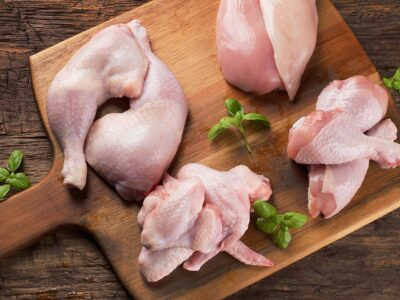Lean Chicken Mince Chicken\$12 jQuery(function(\$){ var price = '12'; currency = '\$'; /* \$('[name=quantity]').change(function(){ if (!(this.value < 1)) { var product_total = parseFloat(price * this.value); \$(this).closest('.woocom-list-content').find('.product_total_price .price .woocommerce-Price-amount').html(currency + product_total.toFixed(2)); } }); jQuery( '.variations_form' ).each( function() { jQuery(this).on( 'found_variation', function( event, variation ) { var price = variation.display_price; var quantity = \$(this).find('[name=quantity]').val(); if (!(quantity < 1)) { console.log(price); var product_total = parseFloat(price * quantity); \$(this).closest('.woocom-list-content').find('.product_total_price .price .woocommerce-Price-amount').html(currency + product_total.toFixed(2)); } }); });*/ }); 500g Lean Chicken Mince quantity ADD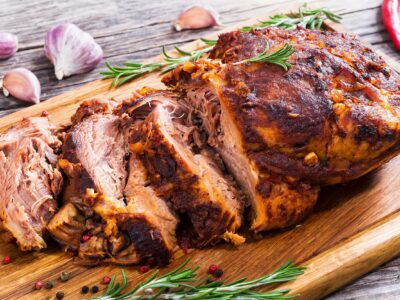Lean Pork Fillet Pork\$15 jQuery(function(\$){ var price = '15'; currency = '\$'; /* \$('[name=quantity]').change(function(){ if (!(this.value < 1)) { var product_total = parseFloat(price * this.value); \$(this).closest('.woocom-list-content').find('.product_total_price .price .woocommerce-Price-amount').html(currency + product_total.toFixed(2)); } }); jQuery( '.variations_form' ).each( function() { jQuery(this).on( 'found_variation', function( event, variation ) { var price = variation.display_price; var quantity = \$(this).find('[name=quantity]').val(); if (!(quantity < 1)) { console.log(price); var product_total = parseFloat(price * quantity); \$(this).closest('.woocom-list-content').find('.product_total_price .price .woocommerce-Price-amount').html(currency + product_total.toFixed(2)); } }); });*/ }); per Kilo400-500g Lean Pork Fillet quantity ADDLean Pork Mince Pork\$15 jQuery(function(\$){ var price = '15'; currency = '\$'; /* \$('[name=quantity]').change(function(){ if (!(this.value < 1)) { var product_total = parseFloat(price * this.value); \$(this).closest('.woocom-list-content').find('.product_total_price .price .woocommerce-Price-amount').html(currency + product_total.toFixed(2)); } }); jQuery( '.variations_form' ).each( function() { jQuery(this).on( 'found_variation', function( event, variation ) { var price = variation.display_price; var quantity = \$(this).find('[name=quantity]').val(); if (!(quantity < 1)) { console.log(price); var product_total = parseFloat(price * quantity); \$(this).closest('.woocom-list-content').find('.product_total_price .price .woocommerce-Price-amount').html(currency + product_total.toFixed(2)); } }); });*/ }); 500g Lean Pork Mince quantity ADD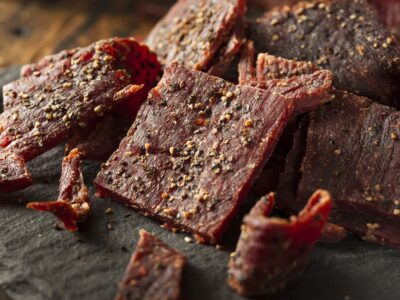Manuka Smoked Cabanossi Dried Meat\$7.00 jQuery(function(\$){ var price = '7.00'; currency = '\$'; /* \$('[name=quantity]').change(function(){ if (!(this.value < 1)) { var product_total = parseFloat(price * this.value); \$(this).closest('.woocom-list-content').find('.product_total_price .price .woocommerce-Price-amount').html(currency + product_total.toFixed(2)); } }); jQuery( '.variations_form' ).each( function() { jQuery(this).on( 'found_variation', function( event, variation ) { var price = variation.display_price; var quantity = \$(this).find('[name=quantity]').val(); if (!(quantity < 1)) { console.log(price); var product_total = parseFloat(price * quantity); \$(this).closest('.woocom-list-content').find('.product_total_price .price .woocommerce-Price-amount').html(currency + product_total.toFixed(2)); } }); });*/ }); A juicy, smoked pork bierstick. These go great as a snack, on a cheese platter or simply diced up and tossed through a salad to give you the edge. 100g Manuka Smoked Cabanossi quantity ADD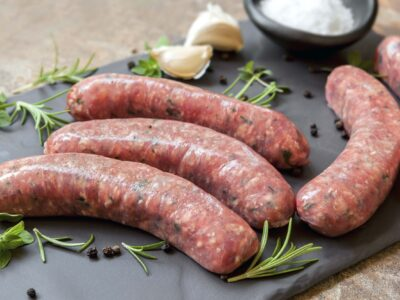Manuka Smoked Cheese Grillers Sausages\$2.9 jQuery(function(\$){ var price = '2.9'; currency = '\$'; /* \$('[name=quantity]').change(function(){ if (!(this.value < 1)) { var product_total = parseFloat(price * this.value); \$(this).closest('.woocom-list-content').find('.product_total_price .price .woocommerce-Price-amount').html(currency + product_total.toFixed(2)); } }); jQuery( '.variations_form' ).each( function() { jQuery(this).on( 'found_variation', function( event, variation ) { var price = variation.display_price; var quantity = \$(this).find('[name=quantity]').val(); if (!(quantity < 1)) { console.log(price); var product_total = parseFloat(price * quantity); \$(this).closest('.woocom-list-content').find('.product_total_price .price .woocommerce-Price-amount').html(currency + product_total.toFixed(2)); } }); });*/ }); Smokey, Cheese Pork Sausage. Manuka Smoked Cheese Grillers quantity ADDManuka Smoked Chorizo Sausages\$2.9 jQuery(function(\$){ var price = '2.9'; currency = '\$'; /* \$('[name=quantity]').change(function(){ if (!(this.value < 1)) { var product_total = parseFloat(price * this.value); \$(this).closest('.woocom-list-content').find('.product_total_price .price .woocommerce-Price-amount').html(currency + product_total.toFixed(2)); } }); jQuery( '.variations_form' ).each( function() { jQuery(this).on( 'found_variation', function( event, variation ) { var price = variation.display_price; var quantity = \$(this).find('[name=quantity]').val(); if (!(quantity < 1)) { console.log(price); var product_total = parseFloat(price * quantity); \$(this).closest('.woocom-list-content').find('.product_total_price .price .woocommerce-Price-amount').html(currency + product_total.toFixed(2)); } }); });*/ }); New Zealand Bronze Medal 2019/2020. A natural Manuka wood smoked spicy Chorizo. Of Spanish/ Portuguese origin. Beautiful in a pasta, on a pizza or straight off the barbie. `chuh~ree~zow` Manuka Smoked Chorizo quantity ADDManuka Smoked Dried Chorizo Dried Meat\$7.00 jQuery(function(\$){ var price = '7.00'; currency = '\$'; /* \$('[name=quantity]').change(function(){ if (!(this.value < 1)) { var product_total = parseFloat(price * this.value); \$(this).closest('.woocom-list-content').find('.product_total_price .price .woocommerce-Price-amount').html(currency + product_total.toFixed(2)); } }); jQuery( '.variations_form' ).each( function() { jQuery(this).on( 'found_variation', function( event, variation ) { var price = variation.display_price; var quantity = \$(this).find('[name=quantity]').val(); if (!(quantity < 1)) { console.log(price); var product_total = parseFloat(price * quantity); \$(this).closest('.woocom-list-content').find('.product_total_price .price .woocommerce-Price-amount').html(currency + product_total.toFixed(2)); } }); });*/ }); Our Bronze Medal New Zealand Award Winning protein snack, a pleasant chill kick with a delicious mouth watering bite. No doubt a top pick for those that enjoy a little spice in their life 100g Manuka Smoked Dried Chorizo quantity ADD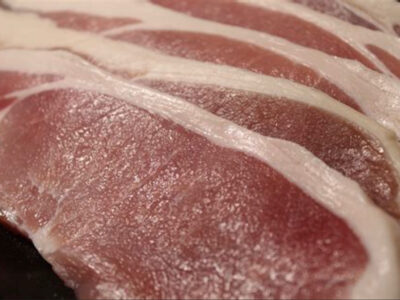Manuka Smoked Middle Bacon Small Goods\$16 jQuery(function(\$){ var price = '16'; currency = '\$'; /* \$('[name=quantity]').change(function(){ if (!(this.value < 1)) { var product_total = parseFloat(price * this.value); \$(this).closest('.woocom-list-content').find('.product_total_price .price .woocommerce-Price-amount').html(currency + product_total.toFixed(2)); } }); jQuery( '.variations_form' ).each( function() { jQuery(this).on( 'found_variation', function( event, variation ) { var price = variation.display_price; var quantity = \$(this).find('[name=quantity]').val(); if (!(quantity < 1)) { console.log(price); var product_total = parseFloat(price * quantity); \$(this).closest('.woocom-list-content').find('.product_total_price .price .woocommerce-Price-amount').html(currency + product_total.toFixed(2)); } }); });*/ }); Free range middle bacon, smoked over a natural Manuka woodfire. An essential for every Sunday morning cooked breakfast. 500g Manuka Smoked Middle Bacon quantity ADD